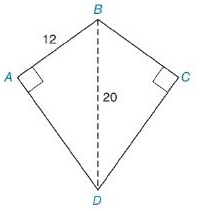Chapter 8.2, Problem 18E### Elementary Geometry for College St...

6th Edition
Daniel C. Alexander + 1 other
ISBN: 9781285195698

#### Solutions

Chapter
Section### Elementary Geometry for College St...

6th Edition
Daniel C. Alexander + 1 other
ISBN: 9781285195698
Textbook Problem
1 views

# In Exercises 13 to 18, find the area of the given polygon.Kite A B C D

To determine

To Find:

Area of the kite.

Explanation

Formula Used:

Pythagorean theorem for the right angle triangle ABC for the hypotenuse AC,

AC2=AB2+BC2.

Calculation:

It is given that the kite ABCD has one of the diagonals BD=d1=20 and the side AB=12 with mBAD=90°, mBCD=90°.

Here the kite is symmetric with respect to the longest diagonal.

### Still sussing out bartleby?

Check out a sample textbook solution.

See a sample solution

#### The Solution to Your Study Problems

Bartleby provides explanations to thousands of textbook problems written by our experts, many with advanced degrees!

Get Started

#### Evaluate the integral. 01/3t21t41dt

Single Variable Calculus: Early Transcendentals, Volume I

#### Convert the expressions in Exercises 6584 to power form. 35x5x8+72x3

Finite Mathematics and Applied Calculus (MindTap Course List)

#### True or False: The function y = f(x) graphed at the right could be a solution to y = x + y.

Study Guide for Stewart's Single Variable Calculus: Early Transcendentals, 8th